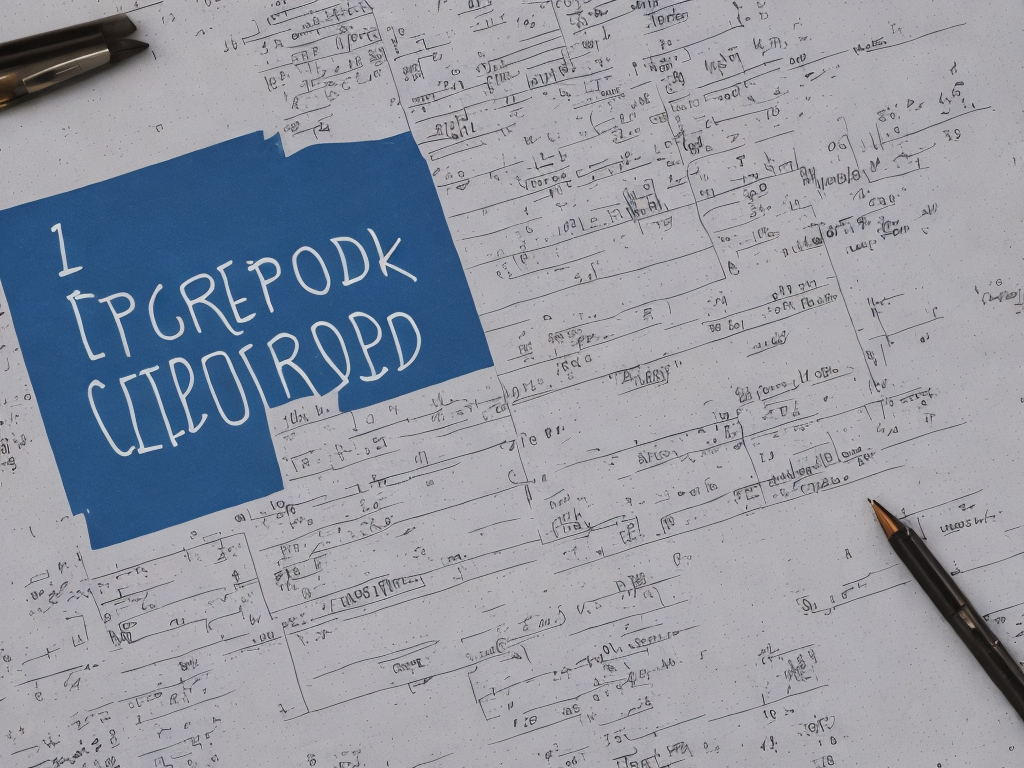# How To Calculate Sslc Percentage

tl;dr
To calculate SSLC percentage, add the marks obtained in all the subjects, divide the total by the maximum marks, and multiply the result by 100.Calculating SSLC percentage can be tricky, but it is an essential aspect for students hoping to secure admission for higher education courses. SSLC or Secondary School Leaving Certificate is the certificate that certifies the completion of secondary education. In India, this certification is issued by the respective State Board of Education after successfully completing the Class 10th Board Examination. The SSLC exam is regarded as one of the most crucial examinations in a student’s life. In India, SSLC Percentage is considered as an essential yardstick of a student’s academic performance. The following text will provide a detailed explanation of how to calculate SSLC percentage.

To calculate SSLC percentage, you first need to know the total marks of each subject in which you were tested. Each State Board of Education in India has its distinctive system of evaluating student’s academic performance. However, a majority of the boards usually conduct written examinations for each subject, which are valued out of a possible 100 or 200 marks.

The SSLC exam consists of various subjects such as Mathematics, Science, English, Social Science, Languages, and other optional subjects that vary from board to board. The total marks or highest marks that can be acquired in each subject varies from board to board. For instance, in some boards, the total marks for Maths is 100, whereas in others, it is 200.

Once you have acquired the total marks of each subject, the next step is to calculate the aggregate percentage. The aggregate percentage is calculated by adding the marks obtained in all the subjects and then dividing the total with the maximum marks and multiplying it by 100. The formula for calculating the aggregate percentage is as follows:

Aggregate Percentage = (Total marks obtained / Maximum marks)*100

For instance, if the total marks that can be scored in Mathematics are 100, and the student has scored 85, the percentage will be calculated as follows:

Percentage = (85 / 100)*100 = 85%

The same formula applies to other subjects in the SSLC examination. Once you have calculated the percentage of each subject, you can then calculate the overall percentage by adding the percentage of each subject and then dividing the total by the number of subjects. The formula for calculating the overall percentage is as follows:

Overall Percentage = (Total Percentage of all subjects / Total number of subjects)

For example, if a student has taken six subjects and their percentage in each subject is as follows:

Maths: 85%

Science: 80%

English: 90%

Social Science: 75%

Hindi: 80%

The total percentage of all subjects will be calculated as follows:

Total Percentage of all subjects = (85+80+90+75+70+80)/6

= 480/6

= 80%

Thus, the overall percentage of the student is 80%.

Another important aspect to note here is that different State Boards of Education in India have varying criteria for passing the SSLC examination. For instance, while some boards ask for a minimum of 35% in each subject, others may require an overall percentage of 35%. Therefore, students are advised to check the criteria set by their respective State Board of Education before attempting to calculate their SSLC percentage.

In conclusion, calculating SSLC percentage requires a bit of effort, but it is a crucial aspect for students looking to pursue higher education. Students should ensure that they have garnered all the necessary information, such as the total marks of each subject and the criteria for passing before attempting to calculate their SSLC percentage. By following the steps mentioned above, students can easily calculate their SSLC percentage and subsequently utilize it for their admission into higher education courses.